Aptitude Tests 4 Me

Download Free EBooks for Various Types of Aptitude Tests

Non Verbal Logic

Detailed solution

106.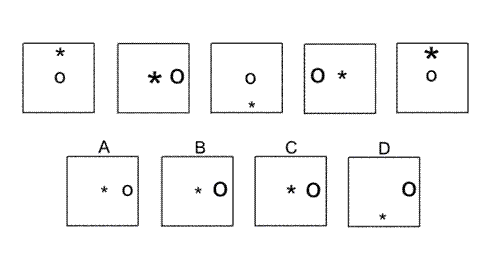Solution: B Explanation: In this question there is an asterisk that has three different sizes (small, medium and large), and a letter O that has two different sizes (small and large). The asterisk moves up and down the vertical axis of symmetry of the square, changing size from medium, to large, to small, back to medium etc The letter O moves backwards and forwards across the horizontal axis of symmetry of the square and alternates between a small O and a large O. Following these rules, for the next diagram of the sequence, there should be a small asterisk in the centre of the square and a large O on the right edge of the square. The correct answer, therefore, is B.

107.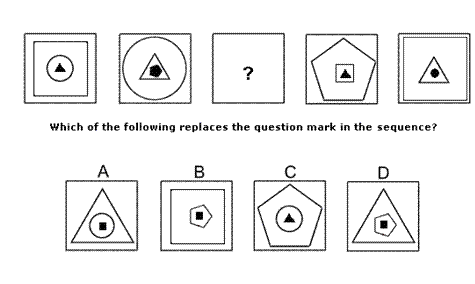Solution: D Explanation: In this question, each diagram has three shapes, one inside the other, with the inner shape coloured black. From one diagram to the next, the middle shape of the three becomes the outer shape, the inner shape becomes the middle shape but is no longer shaded black, and a new black shape is placed in the centre. Following these rules, for the missing diagram of the sequence, the outer shape should be a triangle, the middle shape should be a pentagon and the inner shape should be a black square (the same shape as the middle shape in the fourth diagram). The correct answer, therefore, is D.

108.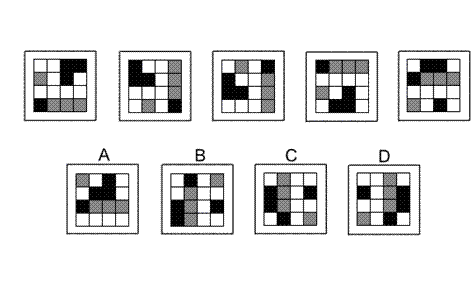Solution: C Explanation: In this question there is a grid of black, grey and white squares. There are two rules to follow that are applied consecutively. The first rule is that the whole diagram is rotated by 90° anticlockwise. The second rule is that the coloured squares all move down the grid one place, with those in the bottom row moving to the top row. The first rule, therefore, should be applied next, and the correct answer is C.

109.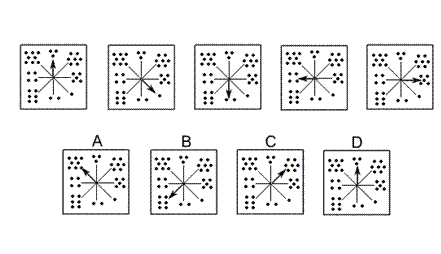Solution: A Explanation: In this question, the arrow moves clockwise around the dial, the number of places it moves being determined by the number of dots from the previous diagram. Following this rule, for the next diagram in the sequence, the arrow should move clockwise by five places, and the correct answer is A.

110.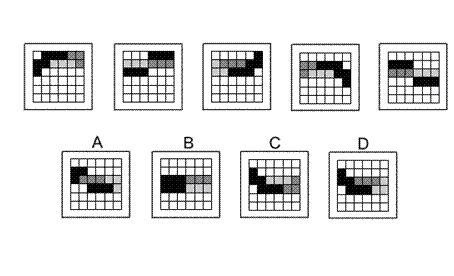Solution: D Explanation: In this question there is a 'snake' of squares consisting from head to tail of four segments – the first with 3 black squares, the second with 3 light grey squares, the third with 3 dark grey squares and the fourth with 3 black squares. The 'snake' starts with its tail in the top row second column and moves around the grid in a zigzag pattern, moving forwards along the zigzag by two places each time. Following this rule, for the next diagram of the sequence, the tail should move two places to the left to be in the second row, first column. Of the two possible answers, C and D, only D has the four segments of the 'snake' in the correct order.

 1 2 3 4 5 6 7 8 9 10 11 12 13 14 15 16 17 18 19 20 21 22 23 24 25 26 27 28 29 30 31 32 33 34 35 36 37 38 39 40 41 42 43 44 45 46 47 48 49 50 51 52 53 54 55 56 57 58 59 60 61 62 63 64 65 66 67 68 69 70 71 72 73 74 75 76 77 78 79 80 81 82 83 84 85 86 87 88Passage ReadingVerbal LogicNon Verbal LogicNumerical LogicData InterpretationReasoningAnalytical AbilityBasic NumeracyAbout UsContactPrivacy PolicyMajor TestsFAQ